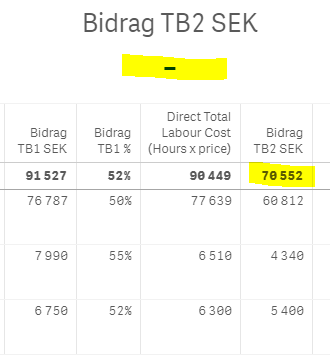# New to Qlik Sense

If you’re new to Qlik Sense, start with this Discussion Board and get up-to-speed quickly.

Announcements
Our May 2021 end-to-end product release from Data Integration to Data Analytics is out! READ DETAILS
cancel
Showing results for
Search instead for
Did you mean:Contributor III

## SUM

Hi!

I have a table that makes a correct Total for TB2, but when use the same field in a KPI I don't get a result!This is my formula:

Num((([Hourly Sales Rate]*[No of Charged Hours])-(([Wage Cost per Hour]+[Overhead Cost per Hour])*[No of Charged Hours])),'# ##0',' ',)

This also works: (([Hourly Sales Rate]*[No of Charged Hours])-(([Wage Cost per Hour]+[Overhead Cost per Hour])*[No of Charged Hours]))

Grateful for help  to get the KPI sum to be the same as the sum in the table.🙂

Labels (3)

• ### sum

1 Solution

Accepted SolutionsMVP

@Go-Anna  you need aggr function here because in KPI you don't have any dimension. try below

=sum(aggr((([Hourly Sales Rate]*[No of Charged Hours])-(([Wage Cost per Hour]+[Overhead Cost per Hour])*[No of Charged Hours])), DimensionofYourTable))

Where DimensionofYourTable is Field used as dimension of your table. If you have multiple dimension then include those as well in aggr like  aggr(........, Dimenion1,Dimension2)

2 RepliesMVP

@Go-Anna  you need aggr function here because in KPI you don't have any dimension. try below

=sum(aggr((([Hourly Sales Rate]*[No of Charged Hours])-(([Wage Cost per Hour]+[Overhead Cost per Hour])*[No of Charged Hours])), DimensionofYourTable))

Where DimensionofYourTable is Field used as dimension of your table. If you have multiple dimension then include those as well in aggr like  aggr(........, Dimenion1,Dimension2)Contributor III
Author

Thank you so much Kush!Tags